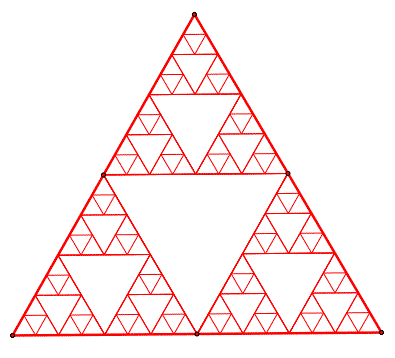# A fractal triangleThe figure above is Sierpinski triangle, It turns out the moment of inertia can be calculated. If the moment of inertial through its center and perpendicular to the plane can be written as $\frac { A }{ B } M{ l }^{ 2 }$. What is the value of $A+B$, where $l$ is the side length and $A$ and $B$ are co-prime positive integers?

Not an original problem

×